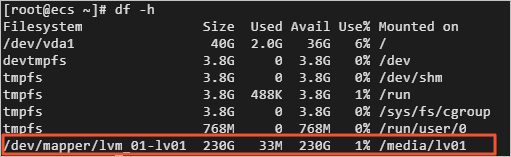This topic describes how to use Logical Volume Manager (LVM) to resize a logical volume (LV) on a Linux Elastic Compute Service (ECS) instance.

## Procedure

1. Connect to an ECS instance as the root user.
2. Run the following command to view information about the LV that is created on the ECS instance:
``lvdisplay``
The following command output indicates that the /dev/lvm_01/lv01 LV is created and has 150 GiB of capacity.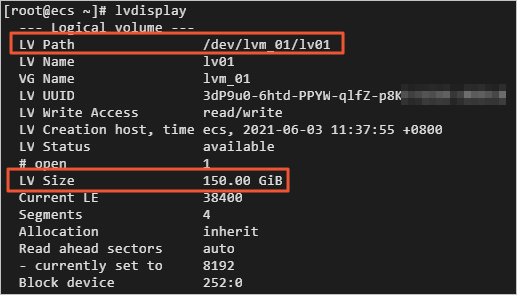3. Run the following command to resize the physical volume (PV) corresponding to the LV:
``pvresize <PV name>``
In this example, the name of the PV is /dev/vdf. In actual application, replace it with the name of your PV.
``pvresize /dev/vdf``
A command output similar to the following one is displayed: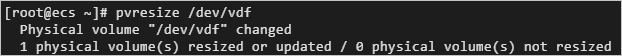4. Run the following command to view the PV usage:
``pvs``
The following command output indicates that the /dev/vdf PV has 80 GiB of free capacity, which consists of the original 40 GiB of capacity and the added 40 GiB of capacity.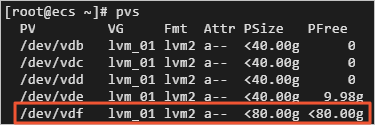5. Run the following command to resize the LV:
``lvextend [-L <LV size>] <LV name>``

In this example, the capacity of the LV is resized.

``lvextend -L +80G /dev/lvm_01/lv01``
The following section describes the variables in this example. You must modify the variables based on your needs.
• `+80GB`: the amount of capacity to increase or decrease. You can resize the LV only when the volume group (VG) has remaining capacity.
• `/dev/lvm_01/lv01`: the name of the LV.
The following command output indicates that you increase the physical capacity by 80 GiB for the /dev/lvm_01/lv01 LV.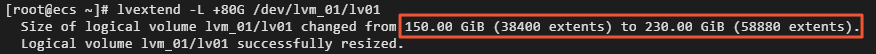6. Run one of the following commands to resize the file system of the LV.
Run one of the following commands based on the file system type of the LV. In these examples, ext4 and xfs file systems are used.
Note If you are uncertain about the file system type of the LV, run the `df -Th` command to query it.
• To resize an ext4 file system, run the following command:
``resize2fs /dev/lvm_01/lv01``
• To resize an xfs file system, run the following command:
``xfs_growfs /dev/lvm_01/lv01``
7. Run the following command to check whether the file system is resized:
``df -h``
In the following command output, the total capacity of the LV is 230 GiB, which indicates that the file system is resized.